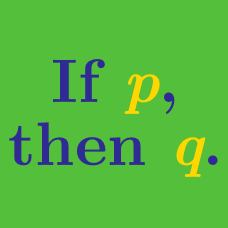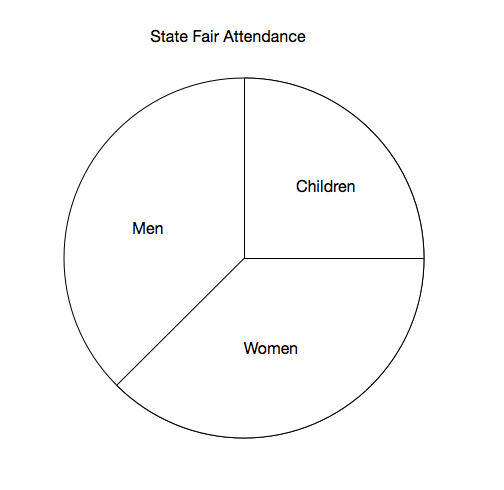Everyday Math

# Estimation

Which of the following is closest to

$123,456,789 \times 987,654,321 ?$

(A) $\ \ 1.0 \times 10^{15}$
(B) $\ \ 1.0 \times 10^{16}$
(C) $\ \ 1.0 \times 10^{17}$
(D) $\ \ 1.0 \times 10^{18}$
(E) $\ \ 1.0 \times 10^{19}$

If Kevin has $25$ quarters, $3$ dimes, $5$ nickels, and $2$ pennies in his pocket, approximately how much money does he have?

(A) $\ \ \2.5$
(B) $\ \ \6.25$
(C) $\ \ \6.8$
(D) $\ \ \25.35$
(E) $\ \ \680$

NOTE: One quarter is $\0.25,$ one dime is $\0.1,$ one nickel is $\0.05,$ and one penny is $\0.01.$

If Jane gets $99$% on her math test, Tom gets $89$% and James gets $71$%, what is their approximate average score?

(A) $\ \ 77$%
(B) $\ \ 80$%
(C) $\ \ 86$%
(D) $\ \ 89$%
(E) $\ \ 94$%The above pie chart shows the number of men, women and children who attended a State Fair. What is the ratio of women to children?

(A) $\ \ 9:4$
(B) $\ \ 4:9$
(C) $\ \ 3:2$
(D) $\ \ 2:3$
(E) $\ \ 1:1$

What is the best approximation of $\sqrt{96}+\sqrt{124}?$

(A) $\ \ 19$
(B) $\ \ 20$
(C) $\ \ 21$
(D) $\ \ 22$
(E) $\ \ 23$

×